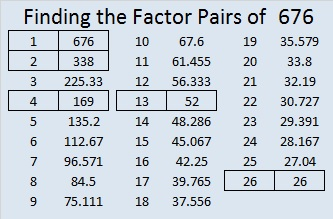# 676 and Level 3

676 is a palindrome in three consecutive different bases plus a couple of other bases:

• 10201 in base 5; note that 1(625) + 0(125) + 2(25) + 0(5) + 1(1) = 676.
• 676 in base 10; note that 6(100) + 7(10) + 6(1) = 676.
• 565 in base 11; note that 5(121) + 6(11) + 5(1) = 676.
• 484 in base 12; note that 4(144) + 8(12) + 4(1) = 676.
• 121 in base 25; note that 1(625) + 2(25) + 1(1) = 676.

Speaking of palindromes, OEIS.org states that 676 is the smallest perfect square palindrome whose square root is not also a palindrome. (Palindromic perfect squares less than 676 are 1, 4, 9, 121, and 484.)

Since 13 and 169 are two of its factors, 676 is also the hypotenuse of Pythagorean triples 476-480-676 and 260-624-676. What is the greatest common factor of each triple?Print the puzzles or type the solution on this excel file: 10 Factors 2015-11-09

—————————————————————————————————

• 676 is a composite number.
• Prime factorization: 676 = 2 x 2 x 13 x 13, which can be written 676 = (2^2) x (13^2)
• The exponents in the prime factorization are 2 and 2. Adding one to each and multiplying we get (2 + 1)(2 + 1) = 3 x 3 = 9. Therefore 676 has exactly 9 factors.
• Factors of 676: 1, 2, 4, 13, 26, 52, 169, 338, 676
• Factor pairs: 676 = 1 x 676, 2 x 338, 4 x 169, 13 x 52, or 26 x 26
• 676 is a perfect square. √676 = 26—————————————————————————————————

A Logical Approach to solve a FIND THE FACTORS puzzle: Find the column or row with two clues and find their common factor. (None of the factors are greater than 10.)  Write the corresponding factors in the factor column (1st column) and factor row (top row).  Because this is a level three puzzle, you have now written a factor at the top of the factor column. Continue to work from the top of the factor column to the bottom, finding factors and filling in the factor column and the factor row one cell at a time as you go.## 2 thoughts on “676 and Level 3”

1.Joseph Nebus

Great set of palindromes. Neat.

•ivasallay

Thank you. It was fun to find them.

This site uses Akismet to reduce spam. Learn how your comment data is processed.